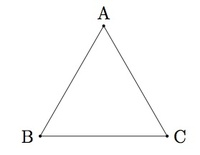# Reflections and Equilateral Triangles

Alignments to Content Standards: G-CO.B

Suppose $ABC$ is an equilateral triangle:1. Describe three reflections of the plane which preserve triangle $ABC$.
2. Show that the three lines of symmetry from part (a) are the perpendicular bisectors of segments $\overline{AB}$, $\overline{AC}$, and $\overline{BC}$.
3. Prove that a triangle $DEF$ is equilateral if and only if there are three reflections of the plane which preserve triangle $DEF$.

## IM Commentary

This activity is one in a series of tasks using rigid transformations of the plane to explore symmetries of classes of triangles, with this task in particular focusing on the class of equilaterial triangles. Students will have previously seen similar material in earlier grades (e.g., standard 4.G.3: "Recognize a line of symmetry for a two-dimensional figure as a line across the figure such that the figure can be folded along the line into matching parts. Identify line-symmetric figures and draw lines of symmetry."), and this task is a similar exploration with a level of mathematical precision more appropriate for high schools students. In particular, the task has students link their intuitive notions of symmetries of a triangle with statements proving that the said triangle is unmoved by applying certain rigid transformations.

The mechanism through which students do this is via congruence proofs, so the task illustrates the general relationship between congruence and rigid transformations, as fits naturally under the cluster "understand congruence in terms of rigid motions." At the high school level, students will need to use the definition of reflections and the teacher may wish to recall this before having students work on the problem. An informal discussion about part (a) may be beneficial as well so that the students have a direction in which to go for part (c) which is more challenging.

If students already know the analogous reflection results for isosceles triangles (see, e.g., the result presented in the task ''Reflections and Isosceles Triangles'') then this task can be accomplished by carefully applying this result three times, as an equilateral triangle is a triangle which can be viewed as an isosceles triangle in three different ways. Students can still work on this problem without prior exposure to ''Reflections and Isosceles Triangles" and an appropriate argument has been supplied. In addition, part (a) requires repeating an argument three times, making it more suitable for group work where students can identify the common argument and not write out the full details three times.

## Solution

1. Since triangle $ABC$ is equilateral, the segments $\overline{AB}$, $\overline{AC}$ and $\overline{BC}$ are all congruent. Corresponding to each of the three pair of congruent sides of $ABC$ is a reflection of the plane which preserves triangle $ABC$ and interchanges the chosen pair congruent sides. These three lines of reflection are

1. the line through $A$ and the midpoint of segment $\overline{BC}$,
2. the line through $B$ and the midpoint of segment $\overline{AC}$,
3. the line through $C$ and the midpoint of segment $\overline{AB}$.

In each case, the reflection preserves triangle $ABC$ provided the line in question is the perpendicular bisector of the appropriate side of the triangle. For convenience, we reproduce here the full argument for case (i). Call $M$ the midpoint of segment $\overline{BC}$ as in the picture below:We wish to show that line $\overleftrightarrow{AM}$ is the perpendicular bisector of $\overline{BC}$. It passes through the midpoint of $\overline{BC}$ so it is sufficient to show that line $\overleftrightarrow{AM}$ is perpendicular to $\overline{BC}$. We will accomplish this by showing that triangles $AMB$ and $AMC$ are congruent. Since angles $AMB$ and $AMC$ make a line and are congruent, they must each be $90$ degree angles. To see why triangles $AMB$ and $AMC$ are congruent, note that segment $\overline{AB}$ is congruent to segment $\overline{AC}$ since triangle $ABC$ is equilateral. Segment $\overline{MB}$ is congruent to segment $\overline{MC}$ because $M$ is the midpoint of $\overline{BC}$. Finally, $\overline{AM}$ is congruent to $\overline{AM}$ and so by SSS triangles $AMB$ and $AMC$ are congruent.

2. The lines from part (a) are the perpendicular bisectors of the three sides $\overline{AB}$, $\overline{AC}$, and $\overline{BC}$ because of the definition of reflections. If a reflection preserves a triangle, it must pass through one vertex and exchange the other two. The reflection which accomplishes this is the one about the perpendicular bisector of the appropriate side of the triangle.

3. The first two parts of this task show that when $DEF$ is an equilateral triangle, there are three reflections of the plane which preserve $DEF$. The only possible reflections of the plane which preserve triangle $DEF$ are the reflections about the perpendicular bisectors of the three sides of the triangle. If all three of these reflections preserve triangle $DEF$ this means that all three sides are congruent: given two sides of the triangle, $\overline{DE}$ and $\overline{DF}$ for example, the reflection about the line joining $D$ and the midpoint of $\overline{EF}$ will map side $\overline{DE}$ to side $\overline{DF}$ and vice versa making these two sides congruent. Arguing in the same way for sides $\overline{DF}$ and $\overline{EF}\,$ will show that they are also congruent and so triangle $DEF$ is equilateral.